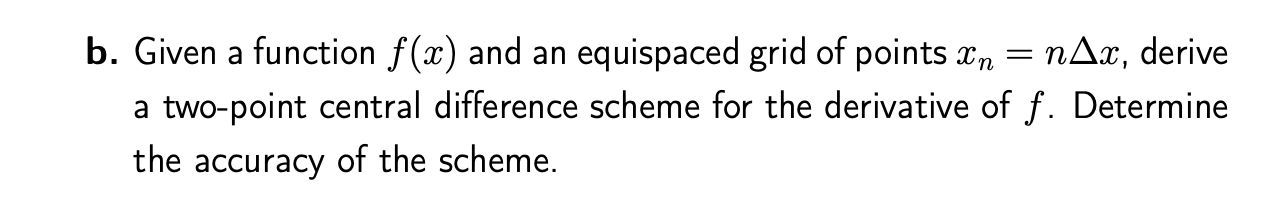Home / Expert Answers / Calculus / what-is-the-detailed-method-for-this-o-given-a-function-f-x-and-an-equispaced-grid-of-poin-pa675

# (Solved): What is the detailed method for this? o. Given a function \( f(x) \) and an equispaced grid of poin ...What is the detailed method for this?

o. Given a function \( f(x) \) and an equispaced grid of points \( x_{n}=n \Delta x \), derive a two-point central difference scheme for the derivative of \( f \). Determine the accuracy of the scheme.

We have an Answer from Expert